[一猫汽车网西安讯]广汽三菱·进口三菱在2018年为消费者量身打造的“高人一筹·帕杰罗超选同行，转动12H”大型试乘试驾&团购会在西安正式拉开帷幕。6月16-17日两天吸引了众多消费者和三菱粉丝们驾临袁家村，与2018款帕杰罗领衔的三菱SUV家族驾享周末派对。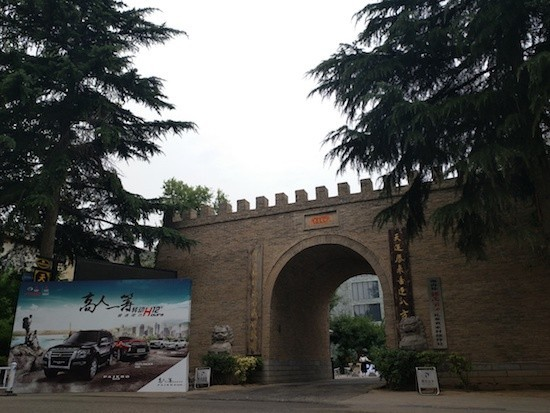活动期间，2018款帕杰罗的“进口高端全路况SUV”全新定位及傲视同级的“七高优势”，迅速得到了消费者的认可。同时，它也是当下越来越多的都市精英们希望选择的一部可满足多种生活方式的全能座驾。而帕杰罗内在的“七高优势”（高视野、高性能、高舒适、高安全、高车身、高贵血统、高性价比）分别映射出了“进取主义”、“创领者”、“细节控”、“稳健派”、“二孩新生活”、“精神贵族”、“务实者”，这七项精英阶层所崇尚的生活方式和价值观，全面彰显了2018款帕杰罗与当下中国汽车消费者的需求高度吻合。

到场即嗨翻的高端团购会率先将活动气氛推向高潮，好礼送不停、优惠无止境的大促销让嘉宾们抢购爱车浪潮一波接一波。随后的试乘试驾在SUV大咖带领下，共同领略“进口高端全路况SUV”全新定位品牌魅力，真切感受到帕杰罗的“七高优势”。最后，在激情驾驭之余，来一场“高人一筹”车尾派对，以及更多家庭休闲活动，充分领悟到帕杰罗为你开启的全新生活方式。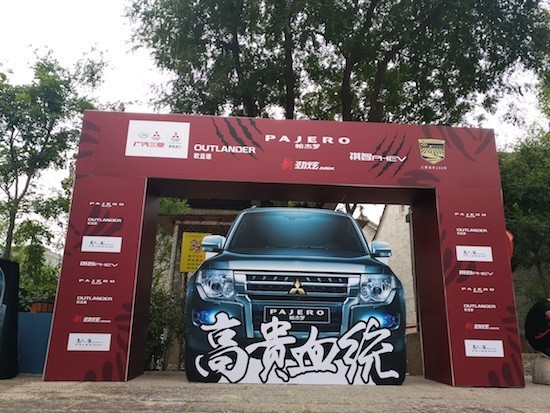出场阵容大牌云集，2018款帕杰罗还满足消费者需求新增一款3.0L自动舒适版车型，配置上介于现有的3.0L自动标准版和豪华版之间。除了感受进口三菱帕杰罗的惊艳表现，消费者还可体验广汽三菱的2018款欧蓝德、2018款劲炫ASX和祺智PHEV, 通过活动对广汽三菱·进口三菱的全系产品有更深的了解。

上午十点左右，我们抵达袁家村。经过全面布置后，这里俨然被打造成了三菱SUV的专属乐园，不仅让到场嘉宾驾车、购车欲望强烈，更感受到了浓郁的三菱品牌文化。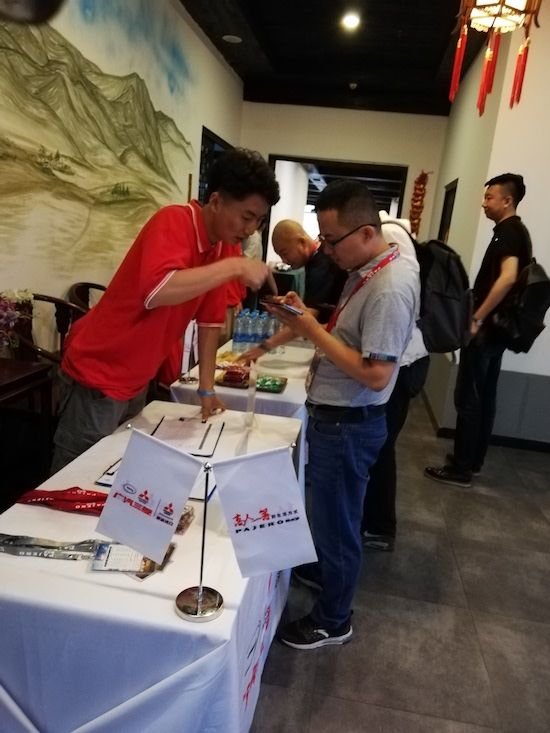嘉宾在现场签到处领取证件后，即可参与“鸿运当头”互动游戏赢得伴手礼。因此，活动气氛从开始就变得热烈起来，让大家对团购会充满更多期待。对于准备购买帕杰罗或三菱其它SUV的消费者来说，本次团购会是出手的最好时机。在主持人的引导下，配合本站活动的厂商和经销商领导也来到现场助兴，亲自为嘉宾颁布诚意十足的购车优惠政策。现场气氛随之变得更加热烈，接下来的抽奖环节一轮接一轮，极高的中奖率让嘉宾们连连叫好。另外，“7高优势有奖问答”、“1元竞拍”、“CALL ME”、“红包任性抢”等寓教于乐的互动游戏同样深受车友们欢迎。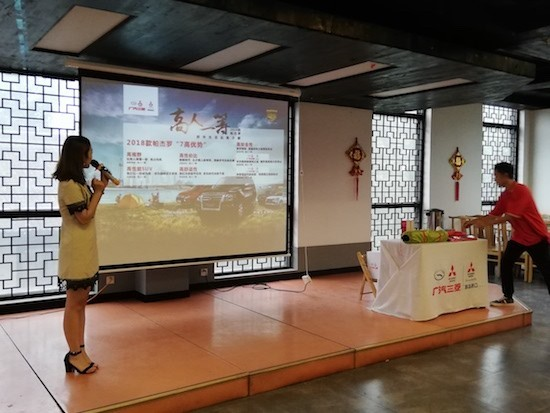团购会结束后，三菱特别准备了当地特色午餐，在尽享美食的同时，车友们的交流更见真情。午餐休息过后，更加精彩的活动随即开始，现场嘉宾除了分组进行超选驾控体验外，还有彰显帕杰罗生活方式的车尾派对和家庭亲子娱乐活动。试驾之前，由资深越野专家主持的大咖讲堂对2018款帕杰罗进行了深入剖析，从品牌定位、产品理念到超凡的七高优势，可谓面面俱到。值得一提是安全驾驶培训和专业越野技巧传授让嘉宾们受益匪浅，对于SUV驾驶技能的提升有很大的帮助。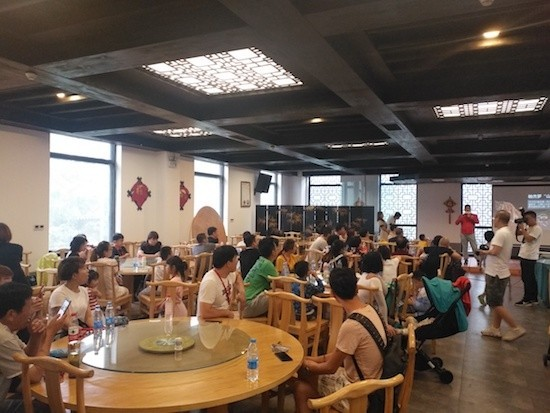帕杰罗作为三菱汽车旗下最为经典的一款车型，配备性能卓越的V6发动机和超选四驱Ⅱ系统，拥有强大的越野性能。同时，“进口高端全路况SUV”全新定位让帕杰罗除了越野性能，更在城市里展现了舒适、豪华、稳重的一面，其对于生活方式的改变与提升是消费者特别看重的。试驾场地复杂的地形凸显了帕杰罗的全地形驾驭能力，其拥有的诸多越野利器：超选四驱、限滑差速器、中后两把锁和内置大梁的承载式车身等让它在越野赛道中表现尤为出色。帕杰罗搭载的3.0升V6发动机，结构简单、故障率低、耐用性强，在低转速驾驶情境下可保证高扭矩输出。在越野路况中，这款V6发动机便能提供可靠、稳定的动力输出。在城市路况中，这款发动机的安静平顺性和燃油经济性也非常突出。帕杰罗历经四代演变，畅销30余年，如今采用的内置梯形结构车架的承载式车身和四轮独立悬挂在硬派越野车中独树一帜，这样的设置不仅让帕杰罗的舒适性提升了一个级别，而且公路上的激烈操控也更随心所欲，让驾驶者在城市道路感受到更多速度与激情。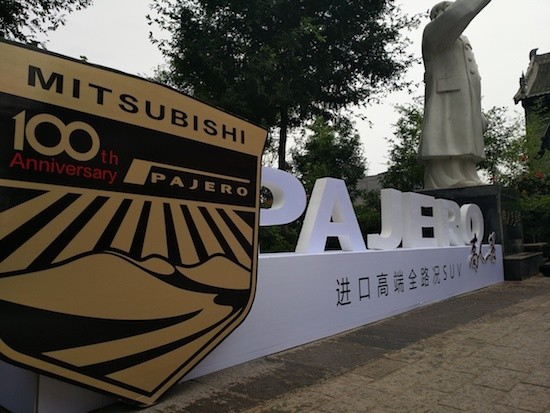试驾体验环节里最刺激的当属“转动中国，超选之王”综合竞技评比。参加活动的消费者要接受多项较有难度的驾驶挑战，并且每一项都会得到试驾教练的评分，最终分数最高的三位嘉宾将获得奖励。迎接挑战的多位选手鼓起勇气，勇闯巅峰，在面对驼峰、沙石、侧坡等地形时表现沉稳，帕杰罗的超强实力让他们信心十足。经过了略显紧张激烈的争夺，表现最佳的三位选手最终站上了领奖台，这一荣耀时刻定会在他们心中记忆很久。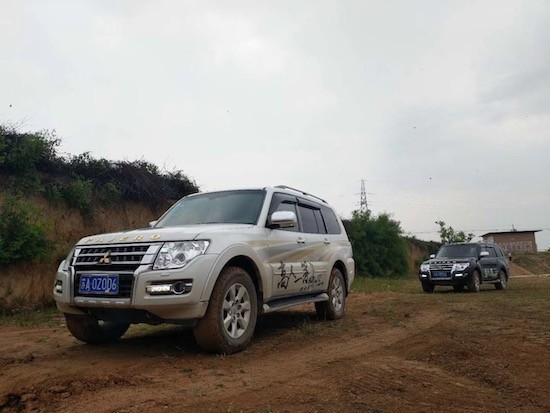除了试驾活动外，本次活动的“高人一筹”车尾派对让嘉宾们在试驾之余更能感受到2018款帕杰罗在都市生活中的另一面，带给车主生活方式的新启发。活动现场特别打造了一片“高人一筹”车尾派对乐享空间，包括3D立体情景展示、露营帐篷展示、生活情景体验等。还设置了包括帕杰罗车尾金库互动在内的三大通关游戏，可通过手机H5完成通关流程赢取丰厚大奖。前来体验的消费者表示打动他们的不只是帕杰罗的产品“七高”，其带来生活方式改变才更加难得。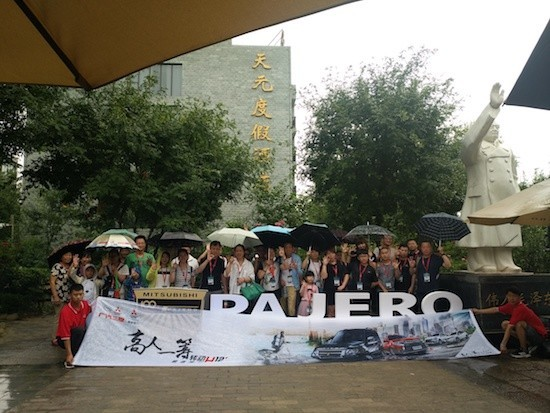高人一筹·帕杰罗超选同行，转动12H已经到来，来参加活动的新老嘉宾以及热衷体验SUV驾驶乐趣的车友们欢聚一堂，与帕杰罗一起驾享周末时光。活动从3月开始一直持续到7月，欢迎广大消费者踊跃报名参加。周末时光因帕杰罗而美好，去品味高端SUV的驾趣生活吧!

##### 相关资讯

|分享

•已阅！握爪
•我手滑为你点赞
•128个赞！
•不明觉厉
•阅后既醉
•有钱！任性
•照片太美，我不敢看
•干货！杠杠的
•高大上
•膜拜中！
•请接受我的膝盖
•猫哥/猫妹么么哒
•神吐槽
•我只笑笑不说话
•我想静静
•窒息
##### 资讯相关车系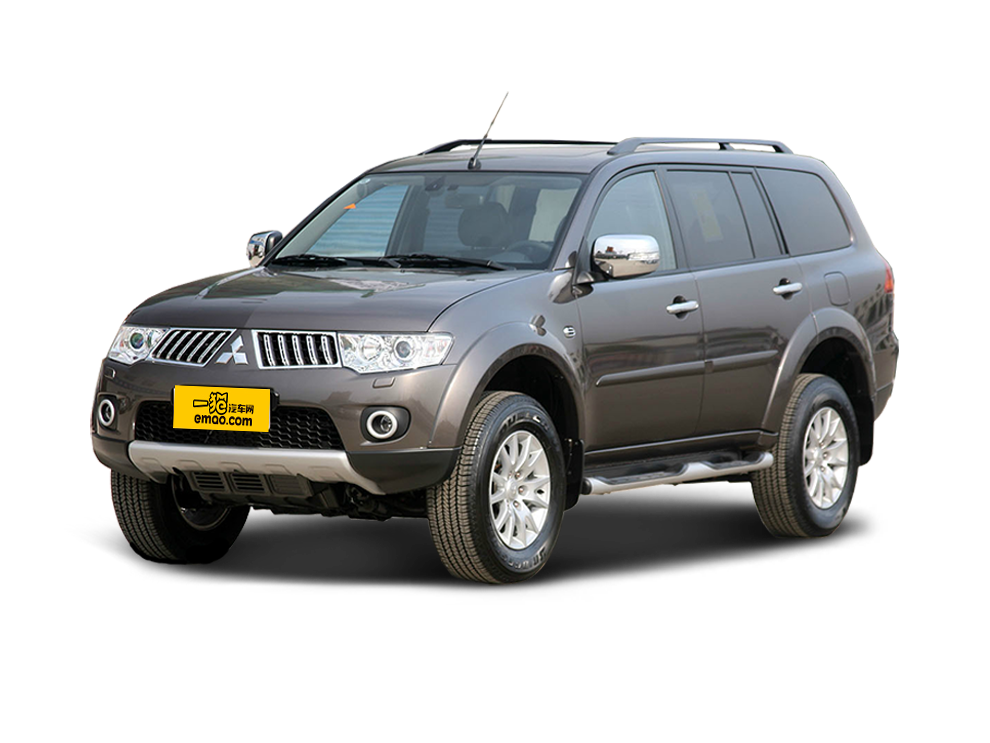##### 热度排行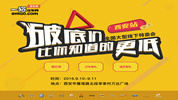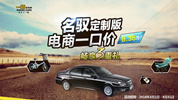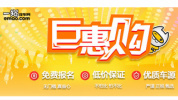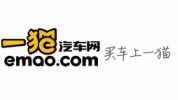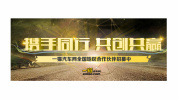• 阿斯顿·马丁
• 奥迪
• 阿尔法罗密欧
• ALPINA

• 巴博斯
• 宝骏
• 宝马
• 保时捷
• 北汽制造
• 奔驰
• 奔腾
• 本田
• 比亚迪
• 标致
• 别克
• 宾利
• 布加迪
• 北汽威旺
• 北京
• 北汽绅宝
• 北汽幻速
• 北汽新能源
• 宝沃
• 比速汽车
• 北汽道达

• 昌河
• 长安
• 长城
• 长安商用
• 成功汽车
• 长江EV
• 长安轻车型

• 大众
• 道奇
• 东风
• 东风风神
• 东风小康
• 东南
• DS
• 东风风行
• 东风风度
• 东风风光

• 法拉利
• 菲亚特
• 丰田
• 福迪
• 福特
• 福田汽车
• 福汽启腾
• 风诺

• GMC
• 广汽传祺
• 广汽吉奥
• 观致

• 哈飞
• 海格
• 海马
• 华泰
• 黄海
• 恒天
• 红旗
• 哈弗
• 华颂
• 华凯
• 华泰新能源
• 汉腾汽车

• Jeep
• 江淮
• 江铃
• 捷豹
• 金杯
• 九龙
• 吉利汽车
• 金旅
• 金龙
• 江铃集团轻汽
• 江铃集团新能源
• 君马汽车
• 捷途

• 开瑞
• 凯迪拉克
• 科尼赛克
• 克莱斯勒
• KTM
• 卡威
• 凯翼
• 康迪
• 康迪电动汽车集团

• 猎豹汽车
• 兰博基尼
• 劳斯莱斯
• 雷克萨斯
• 雷诺
• 理念
• 力帆
• 莲花汽车
• 林肯
• 铃木
• 陆风
• 路虎
• 路特斯
• 领志
• 领克
• 零跑汽车

• MG
• MINI
• 马自达
• 玛莎拉蒂
• 迈凯伦
• 摩根

• 纳智捷

• 讴歌
• 欧宝
• 欧朗
• 欧拉
• 欧尚汽车

• 帕加尼

• 奇瑞
• 启辰
• 起亚
• 前途
• 庆铃汽车

• 日产
• 荣威
• 瑞麒汽车
• 如虎
• 瑞驰

• 上汽大通
• smart
• 三菱
• 双环
• 双龙
• 斯巴鲁
• 斯柯达
• 萨博
• 思铭
• 赛麟
• SWM斯威汽车

• TESLA
• 泰卡特
• 腾势

• 威麟
• 威兹曼
• 沃尔沃
• 五菱汽车
• 五十铃
• 潍柴英致
• WEY
• 蔚来
• 威马汽车

• 现代
• 雪佛兰
• 雪铁龙
• 西雅特
• 新特汽车
• 小鹏汽车
• 新宝骏

• 野马汽车
• 一汽
• 依维柯
• 英菲尼迪
• 永源
• 驭胜

• 中华
• 中兴
• 众泰
• 知豆
• 之诺
• 正道汽车
• A
• B
• C
• D
• E
• F
• G
• H
• I
• J
• K
• L
• M
• N
• O
• P
• Q
• R
• S
• T
• U
• V
• W
• X
• Y
• Z

• 阿斯顿·马丁
• 奥迪
• 阿尔法罗密欧
• ALPINA

• 巴博斯
• 宝骏
• 宝马
• 保时捷
• 北汽制造
• 奔驰
• 奔腾
• 本田
• 比亚迪
• 标致
• 别克
• 宾利
• 布加迪
• 北汽威旺
• 北京
• 北汽绅宝
• 北汽幻速
• 北汽新能源
• 宝沃
• 比速汽车
• 北汽道达

• 昌河
• 长安
• 长城
• 长安商用
• 成功汽车
• 长江EV
• 长安轻车型

• 大众
• 道奇
• 东风
• 东风风神
• 东风小康
• 东南
• DS
• 东风风行
• 东风风度
• 东风风光

• 法拉利
• 菲亚特
• 丰田
• 福迪
• 福特
• 福田汽车
• 福汽启腾
• 风诺

• GMC
• 广汽传祺
• 广汽吉奥
• 观致

• 哈飞
• 海格
• 海马
• 华泰
• 黄海
• 恒天
• 红旗
• 哈弗
• 华颂
• 华凯
• 华泰新能源
• 汉腾汽车

• Jeep
• 江淮
• 江铃
• 捷豹
• 金杯
• 九龙
• 吉利汽车
• 金旅
• 金龙
• 江铃集团轻汽
• 江铃集团新能源
• 君马汽车
• 捷途

• 开瑞
• 凯迪拉克
• 科尼赛克
• 克莱斯勒
• KTM
• 卡威
• 凯翼
• 康迪
• 康迪电动汽车集团

• 猎豹汽车
• 兰博基尼
• 劳斯莱斯
• 雷克萨斯
• 雷诺
• 理念
• 力帆
• 莲花汽车
• 林肯
• 铃木
• 陆风
• 路虎
• 路特斯
• 领志
• 领克
• 零跑汽车

• MG
• MINI
• 马自达
• 玛莎拉蒂
• 迈凯伦
• 摩根

• 纳智捷

• 讴歌
• 欧宝
• 欧朗
• 欧拉
• 欧尚汽车

• 帕加尼

• 奇瑞
• 启辰
• 起亚
• 前途
• 庆铃汽车

• 日产
• 荣威
• 瑞麒汽车
• 如虎
• 瑞驰

• 上汽大通
• smart
• 三菱
• 双环
• 双龙
• 斯巴鲁
• 斯柯达
• 萨博
• 思铭
• 赛麟
• SWM斯威汽车

• TESLA
• 泰卡特
• 腾势

• 威麟
• 威兹曼
• 沃尔沃
• 五菱汽车
• 五十铃
• 潍柴英致
• WEY
• 蔚来
• 威马汽车

• 现代
• 雪佛兰
• 雪铁龙
• 西雅特
• 新特汽车
• 小鹏汽车
• 新宝骏

• 野马汽车
• 一汽
• 依维柯
• 英菲尼迪
• 永源
• 驭胜

• 中华
• 中兴
• 众泰
• 知豆
• 之诺
• 正道汽车
• A
• B
• C
• D
• E
• F
• G
• H
• I
• J
• K
• L
• M
• N
• O
• P
• Q
• R
• S
• T
• U
• V
• W
• X
• Y
• Z

• 阿斯顿·马丁
• 奥迪
• 阿尔法罗密欧
• ALPINA

• 巴博斯
• 宝骏
• 宝马
• 保时捷
• 北汽制造
• 奔驰
• 奔腾
• 本田
• 比亚迪
• 标致
• 别克
• 宾利
• 布加迪
• 北汽威旺
• 北京
• 北汽绅宝
• 北汽幻速
• 北汽新能源
• 宝沃
• 比速汽车
• 北汽道达

• 昌河
• 长安
• 长城
• 长安商用
• 成功汽车
• 长江EV
• 长安轻车型

• 大众
• 道奇
• 东风
• 东风风神
• 东风小康
• 东南
• DS
• 东风风行
• 东风风度
• 东风风光

• 法拉利
• 菲亚特
• 丰田
• 福迪
• 福特
• 福田汽车
• 福汽启腾
• 风诺

• GMC
• 广汽传祺
• 广汽吉奥
• 观致

• 哈飞
• 海格
• 海马
• 华泰
• 黄海
• 恒天
• 红旗
• 哈弗
• 华颂
• 华凯
• 华泰新能源
• 汉腾汽车

• Jeep
• 江淮
• 江铃
• 捷豹
• 金杯
• 九龙
• 吉利汽车
• 金旅
• 金龙
• 江铃集团轻汽
• 江铃集团新能源
• 君马汽车
• 捷途

• 开瑞
• 凯迪拉克
• 科尼赛克
• 克莱斯勒
• KTM
• 卡威
• 凯翼
• 康迪
• 康迪电动汽车集团

• 猎豹汽车
• 兰博基尼
• 劳斯莱斯
• 雷克萨斯
• 雷诺
• 理念
• 力帆
• 莲花汽车
• 林肯
• 铃木
• 陆风
• 路虎
• 路特斯
• 领志
• 领克
• 零跑汽车

• MG
• MINI
• 马自达
• 玛莎拉蒂
• 迈凯伦
• 摩根

• 纳智捷

• 讴歌
• 欧宝
• 欧朗
• 欧拉
• 欧尚汽车

• 帕加尼

• 奇瑞
• 启辰
• 起亚
• 前途
• 庆铃汽车

• 日产
• 荣威
• 瑞麒汽车
• 如虎
• 瑞驰

• 上汽大通
• smart
• 三菱
• 双环
• 双龙
• 斯巴鲁
• 斯柯达
• 萨博
• 思铭
• 赛麟
• SWM斯威汽车

• TESLA
• 泰卡特
• 腾势

• 威麟
• 威兹曼
• 沃尔沃
• 五菱汽车
• 五十铃
• 潍柴英致
• WEY
• 蔚来
• 威马汽车

• 现代
• 雪佛兰
• 雪铁龙
• 西雅特
• 新特汽车
• 小鹏汽车
• 新宝骏

• 野马汽车
• 一汽
• 依维柯
• 英菲尼迪
• 永源
• 驭胜

• 中华
• 中兴
• 众泰
• 知豆
• 之诺
• 正道汽车
• A
• B
• C
• D
• E
• F
• G
• H
• I
• J
• K
• L
• M
• N
• O
• P
• Q
• R
• S
• T
• U
• V
• W
• X
• Y
• Z